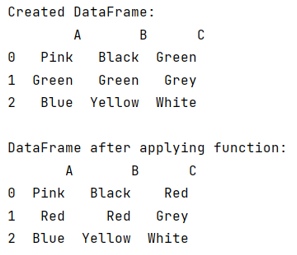# Apply function to each cell in DataFrame

Given a Pandas DataFrame, we have to apply function to each cell.
Submitted by Pranit Sharma, on July 01, 2022

Pandas is a special tool that allows us to perform complex manipulations of data effectively and efficiently. Inside pandas, we mainly deal with a dataset in the form of DataFrame. DataFrames are 2-dimensional data structures in pandas. DataFrames consist of rows, columns, and data. A certain operation can be performed on DataFrames.

## Problem statement

Given a Pandas DataFrame, write a function and apply it on each cell of the given DataFrame.

## Apply function to each cell in DataFrame

To apply function to each cell in DataFrame, we will first define a function that we want to apply to each cell and then we will use np.vectorize() method which will be useful for applying the function to each cell, the function which we want to apply to each cell will be pasted inside this method as a parameter.

Note

To work with pandas, we need to import pandas package first, below is the syntax:

```import pandas as pd
```

Let us understand with the help of an example,

## Python program to apply function to each cell in DataFrame

```# Importing pandas package
import pandas as pd

# Importing numpy package
import numpy as np

# Creating a DataFrame
df = pd.DataFrame({
'A':['Pink','Green','Blue'],
'B':['Black','Green','Yellow'],
'C':['Green','Grey','White']
})

# Display DataFrame
print("Created DataFrame:\n",df,"\n")

# Defining a function
def function(x):
return x.replace('Green','Red')

# Applying function to all the cells
result = df.apply(np.vectorize(function))

# Display result
print("DataFrame after applying function:\n",result)
```

## Output

The output of the above program is: